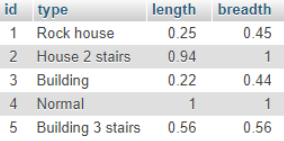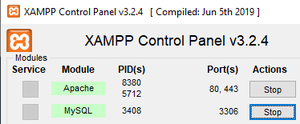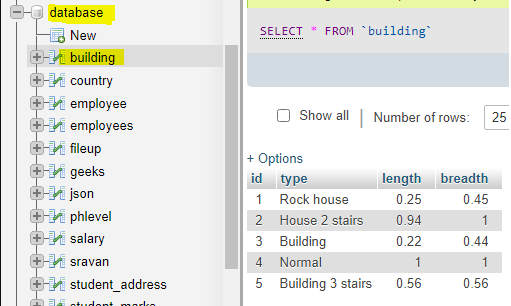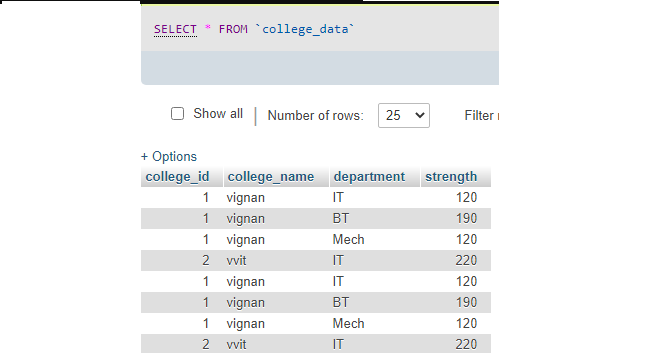Related Articles

# How to count rows in MySQL table in PHP ?

• Last Updated : 26 May, 2021

PHP stands for hypertext preprocessor. MySQL is a database query language used to manage databases.

In this article, we are going to discuss how to get the count of rows in a particular table present in the database using PHP and MySQL.

Requirements:

Approach: By using PHP and MySQL, one can perform database operations. We can get the total number of rows in a table by using the MySQL mysqli_num_rows() function.

Syntax:

mysqli_num_rows( result );

The result is to specify the result set identifier returned by mysqli_query() function.

Example: The following table has 5 rows.To count the number of rows in the building table, the following code snippet is used.

```\$sql = "SELECT * from building";

if (\$result = mysqli_query(\$con, \$sql)) {

// Return the number of rows in result set
\$rowcount = mysqli_num_rows( \$result );

// Display result
printf("Total rows in this table :  %d\n", \$rowcount);
}```

Output: The expected result is as follows.

`Total rows in this table : 5`

Steps for the approach:

• Create a database named database.
• Create a table named building inside the database.
• Insert records into it.
• Write PHP code to count rows.

Steps:

• Start XAMPP server.XAMPP server

• Create a database named database and create a table named building inside the database.
• Insert records into itbuilding table

• Write PHP code to count rows.

PHP code:

## PHP

 ``

Output: After running the above PHP file in localhost, the following result is achieved.

`Total rows in this table : 5`

Example 2: In the following example, we count the table rows using MySQL count() function. It’s an aggregate function used to count rows.

Syntax:

select count(*) from table;

Consider the table.PHP code:

## PHP

 `query(``\$sql``);`` ` `// Display data on web page``while``(``\$row` `= mysqli_fetch_array(``\$result``)) {``    ``echo` `"Total Rows is "``. ``\$row``[``'count(*)'``];``    ``echo` `"
"``;``}``  ` `// Close the connection``\$conn``->close();`` ` `?>`

Output:

`Total Rows is 8`

My Personal Notes arrow_drop_up